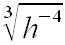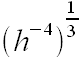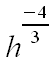SEARCH HOMEMath Central Quandaries & QueriesQuestion from Pete, a student: Hi, How do you express ³√h-4 in exponential form. I am having a lot of trouble with this one. thanks PeteHi Pete. It looks like you wrote this:The radical sign with a 3 in the crook means cube root. That means the same as the exponent of 1/3.Remember that with rules of exponents, you multiply the exponents together to simplify from this form:Hope this helps,
Stephen La Rocque.Math Central is supported by the University of Regina and The Pacific Institute for the Mathematical Sciences.